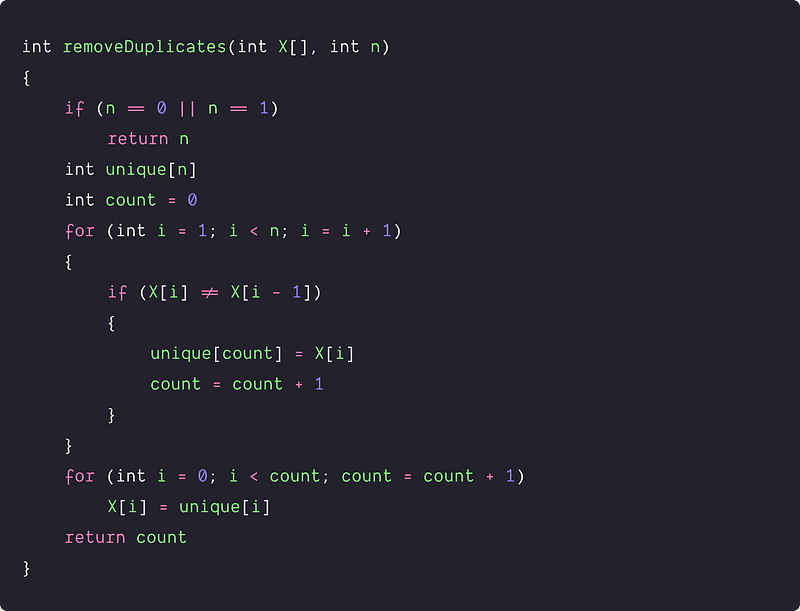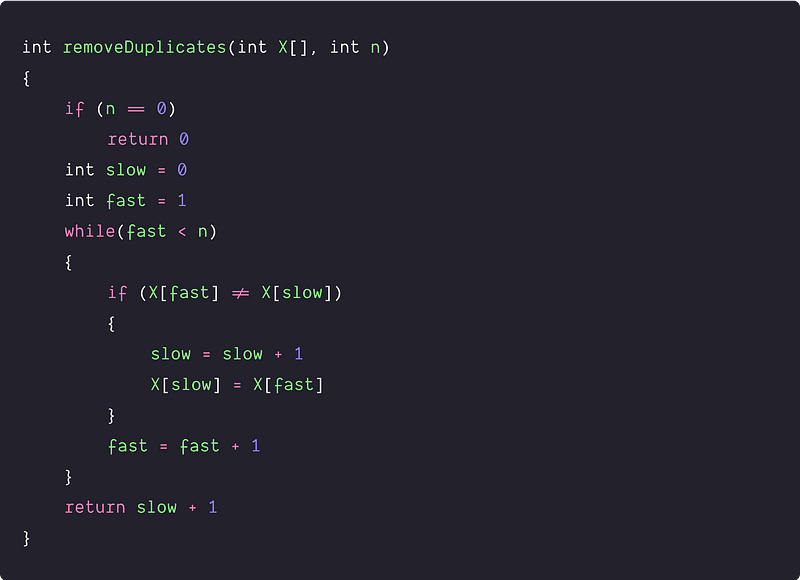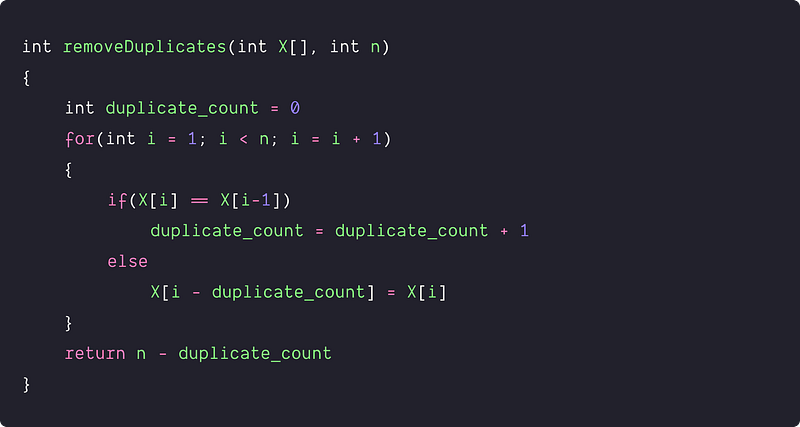# Remove duplicates from sorted array

Key takeaway: A famous modification problem to learn optimization using fast and slow pointers. Here we have discussed two O(n) in-place solutions.

### Let’s understand the problem

We are given a sorted array, write a program to remove the duplicate elements such that there is a single occurrence of each element in the array.

• We need to return the length of the array containing unique elements. In other words: there is no need to delete the unnecessary elements at the end after removing the duplicates. It doesn't matter what you leave beyond the new length.
• The Input array is passed in by reference, which means a modification to the input array will be known to the caller.

Example 1

Input: X[] = [2, 2, 2, 2, 2, 3, 3, 3], Output: 2, X[] = [2, 3]

Explanation: Our function should return length = 2, with the first two elements of X[] being 2 and 3 respectively. It doesn't matter what we leave beyond the returned length.

Example 2

Input: X[] = [1, 2, 2, 3, 4, 4, 4, 5, 5], Output: 5, X[] = [1, 2, 3, 4, 5]

Explanation: Our function should return length = 5, with the first five elements of X[] being 1, 2, 3, 4 and 5 respectively.

### Discussed Solution Approaches

1. A brute force approach using extra memory
2.  An efficient approach using two pointers
3.  An efficient approach by counting the duplicates

### A brute force approach using extra memory

Solution Idea and Steps

One idea would be to store the unique elements into the extra memory and copy the unique elements to the original array. By the end of this process, we return the new length of the input array.

1. We declare extra array unique[n] to store unique elements.
2. Now we scan the input array and copy unique elements of X[] to unique[]. We will keep track of the unique element count using the variable count.
3. Now we copy unique elements from unique[] to X[].
4. Finally, we return the value of the count.

Solution pseudocodeTime and space complexity analysis

We are linearly traversing the array twice. So time complexity = Traversing the array to store the unique elements + Traversing the array to copy the unique elements = O(n) + O (count) = O(n). The value of count is equal to n in the worst case (Think!). Space complexity = O(n) for the extra array unique[n].

### An efficient approach using two pointers

Solution Idea

Can we use the sorted array property to solve this problem in place? Let's think.

In the sorted array, all duplicate elements will be placed adjacent to each other. So, we can easily track the unique elements and place them into the front positions of the array. For this purpose, we use one fast pointer to scan the array and the slow pointer to track the position of the unique elements.

Solution Steps

• We initialise the slow and fast pointers i.e. slow = 0, fast = 1
• Now scan the input array till fast < n. Whenever we find a duplicate element i.e X[fast] == X[slow], then we skip the duplicate and increment the fast by 1. But when we encounter a unique element i.e. X[fast] != X[slow], then we increment the slow by 1 and copy X[fast] to X[slow]. We also increment the fast by 1.
• We repeat the above process until fast reaches the end of the array. Finally, we return the count of the unique element which is slow + 1. (Think!)

Solution PseudocodeTime and space complexity analysis

We are doing a single scan of the array, so time complexity = O(n). We are just using two extra variables, Space complexity = O(1).

### An efficient approach by counting the duplicates

Solution Idea and Steps

Here is another idea to solve the problem by counting the duplicate in the array.

• We scan the array and track the duplicate element count using the variable duplicate_count.
• When we found a duplicate i.e. X[i] == X[i-1], then we increase the variable duplicate_count by one.
• Otherwise, we find the first appearance of the unique element and we place the unique element X[i] at the front i.e. at index i  -  duplicate_count.
• Finally, we return the unique elements count i.e. n  -  duplicate_count. (Think!)

Solution PseudocodeTime and space complexity analysis

We are doing a single scan of the array, so time complexity = O(n). Space complexity = O(1), we are just using one extra variable.

### Critical ideas to think!

• What would be the best and worst-case scenario of all three approaches?
• Will the above solutions work when there are no duplicate elements at all?
• Inside the while loop of the 2nd approach, if (X[fast] != X[slow]), why are we incrementing the slow pointer before copying the value? Similarly in the 3rd approach, if (X[i] == X[i-1]), why are we copying X[i] at X[ i - duplicate_count]?
• Can we solve this problem using BST or other data structures?
• To solve this problem, can we use an idea similar to the quicksort partition process?
• Why does the two-pointers approach work perfectly in the case of a sorted array?
• How do we approach this problem if we have an unsorted array in the input?

### Comparisons of time and space complexities

• Brute force approach: Time = O(n), Space = O(n)
• Using two pointers: Time = O(n), Space = O(1)
• By counting duplicates: Time = O(n), Space = O(1)

### Similar coding questions to practice

• Sort an array of 0s, 1s and 2s
• Move zeroes to an end
• Sort an array in a waveform
• Find duplicates in an Array with values 1 to N
• Valid Mountain in an array
• Find the frequencies of all duplicates elements in the array
• Remove the duplicates such that duplicates appeared at most twice

Thanks to Suaysh Namdeo for his contribution in creating the first version of the content. Please write comments if you find an error or you want to share more insights about the topic. Enjoy learning, Enjoy coding, Enjoy algorithms!

Get well-designed application and interview centirc content on ds-algorithms, machine learning, system design and oops. Content will be delivered weekly.